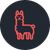# Calculate field not updating after re open from draft

335
2
05-22-2022 02:30 PMNew Contributor

Survey with multiple calculate fields in the form of coalesce(\${no_algae_1},0) + coalesce(\${film_1},0) + coalesce(\${sludge_1},0) + coalesce(\${cyanobacteria_1},0) + coalesce(\${didymo_1},0)+ coalesce(\${other_mats_1},0) + coalesce(\${green_filaments_1},0) + coalesce(\${other_filaments_1},0) +coalesce( \${other_1},0).

All works ok  until the form is saved as draft and then re opened. The calculate fields then no longer update/calculate as changes are made to the associated fields.

I have a work around in that on my form I am able to hide and then redisplay all the fields. When re displayed all the fields have been emptied. As I then fill them in again the calculate field does work.

All fields are integers.

Seen on version 3.14.255, but also then demonstrated on 3.14.242.

1 Solution

Accepted SolutionsbyEsri Contributor

Have you tried setting the calculation mode to always?

The calculationMode parameter entered in the bind::esri:parameters column controls when calculations in questions are calculated, for example, calculationMode=manual. There are three supported values:

• auto—Calculate when the question is empty or has not been previously modified by the user. If no value is provided, this is the default calculation behavior.
• manual—The question will only calculate when clicking the Calculate button next to the question.
• always—Calculate when any value referenced in the calculation expression changes. This includes dynamically changing functions, such as now().

Help Documentation

- Jen
2 RepliesbyEsri Contributor

Have you tried setting the calculation mode to always?

The calculationMode parameter entered in the bind::esri:parameters column controls when calculations in questions are calculated, for example, calculationMode=manual. There are three supported values:

• auto—Calculate when the question is empty or has not been previously modified by the user. If no value is provided, this is the default calculation behavior.
• manual—The question will only calculate when clicking the Calculate button next to the question.
• always—Calculate when any value referenced in the calculation expression changes. This includes dynamically changing functions, such as now().

Help Documentation

- JenNew Contributor

This did fix the issue.

But I find the behaviour inconsistent from the documentation:
# Integer values continue calculating until we save to draft and re open
# Decimal values continue calculating whether in original form or a re opened draft.

But date and time only calculate once which is very good - definitely what we need.

I applied the fix to integer and decimal calculations just in case anther application update modifies the behaviour to remove the inconsistencies.